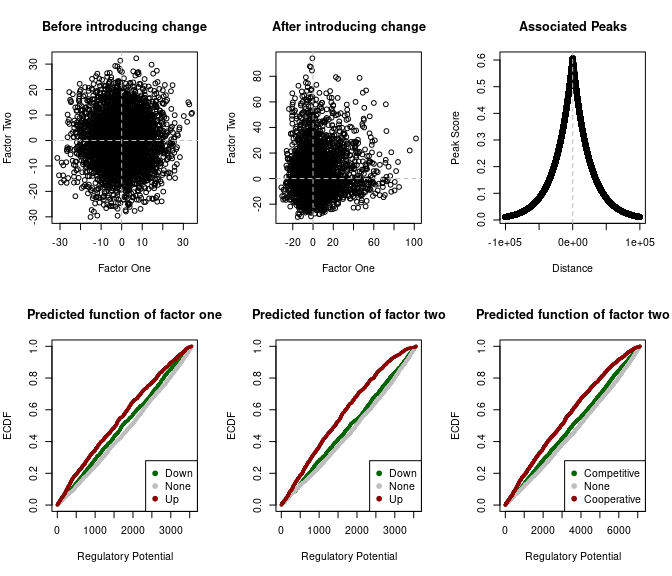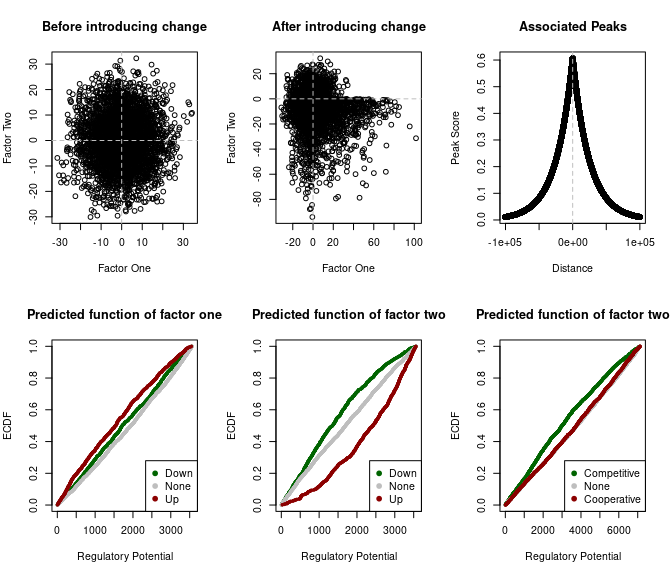# Using target to predict combined binding

## Overview

In this document, we extend the BETA algorithm to predict cooperative and/or competitive binding events of two factors at the same region of interest. This method uses the same theory described in vignette('target') with one modification. Instead of using an individual statistics from a factor perturbation experiment, we use two separate statistics from two comparable factor perturbation experiments to define a regulatory interaction ($$RI$$). This regulatory interaction terms are used to calculate rank products of the regions of interest. In addition, we illustrate the use of this method with a simulated dataset and an example of real-world data.

## Theory

This method is an extension of the BETA basic algorithm. Briefly, the rank product of a regions of interest ($$RP_g$$) is the product of two terms; the rank of a statistics from factor perturbation experiment ($$R_{ge}$$) and the rank of the regulatory potential ($$R_{gb}$$). The regulatory potential of region of interest ($$S_g$$) is the sum of the peaks within a predefined distance:

$S_g = \sum_{i=1}^k e^{-(0.5+4\Delta_i)} \quad\text{and}\quad RP_g = \frac{R_{gb}\times R_{ge}}{n^2}$ Where $$p$$ is a peak in $$\{1,...,k\}$$ with a distance, $$\Delta$$, from the center of the region of interest.

Two determine the relation of two factors $$x$$ and $$y$$ on a common peak near a region of interest, we define a new term; the regulatory interaction ($$RI$$) as the product of two signed statistics from comparable perturbation experiments. The rank of this term is used to calculate a rank product ($$PR_g$$) for each region of interest as described above

$RI_{g} = x_{ge}\times y_{ge} \quad\text{and}\quad RP_g = \frac{R_{gb}\times RI_{ge}}{n^2}$

This term would represent the interaction magnitude assuming a linear relation between the two factor. The sign of the term would define the direction of the relation were positive means cooperative and negative means competitive.

## Example

The target package contain two simulated datasets. sim_peaks is random peaks with random distances from the transcripts of chromosome 1 of the mm10 mouse genome. sim_transcripts is the same transcripts with random singed statistics assigned to each. In the following two examples, we introduce changes in these statistics to simulate conditions where two factors are working cooperatively or competitively on the same transcripts.

# load libraries
library(target)
# load data
data("sim_peaks")
data("sim_transcripts")

To help visualize these cases, a plotting function plot_profiles was constructed to introduce the changes change in the statistics of the transcripts near the n number of peaks. The source code for the function is available in inst/extdata/plot-profiles.R which we source to use here. The output of the function is a series of plots to visualize the statistics of the two factors before and after introducing the changes, the peaks distances and scores and the predicted functions of the factors individually and combined.

# source the plotting function
source(system.file('extdata', 'plot-profiles.R', package = 'target'))

### Cooperative factors example

The first two inputs to the plotting function is the simulated peaks and transcripts. We chose to introduce positive changes to the statistics of the transcripts with the top 5000 nearby peaks of the two factors.

# simulate and plot cooperative factors
plot_profiles(sim_peaks,
sim_transcripts,
n = 5000,
change = c(3, 3))The changes introduced above are illustrated in the right upper quadrant of the scatter plot. The predicted functions of the two factors are similar, as shown by distribution function of the regulatory potential of their targets. Finally, when the targets are predicted based on the two statistics combined, the sign of the statistics product determines the direction of the factor interactions. Here, more higher ranking transcripts had positive/red/ cooperative change associated with the two factors.

### Competitive factors example

Similar to the example above, we chose to introduce change to the statistics of the transcripts with nearby peaks for the two factors. To simulate the competitive conditions of the two factors, the changes have opposite signs.

# simulate and plot competitve factors
plot_profiles(sim_peaks,
sim_transcripts,
n = 5000,
change = c(3, -3))By contrast, the changes associated with each factor are opposite in sign, as shown in the lower right quadrant. The predicted functions of the individual factors are also the opposite. The predicted combined effect of the two factors is negative/green/competitive.